Examples

Chapter 15 Class 11 Statistics (Term 1)
Serial order wise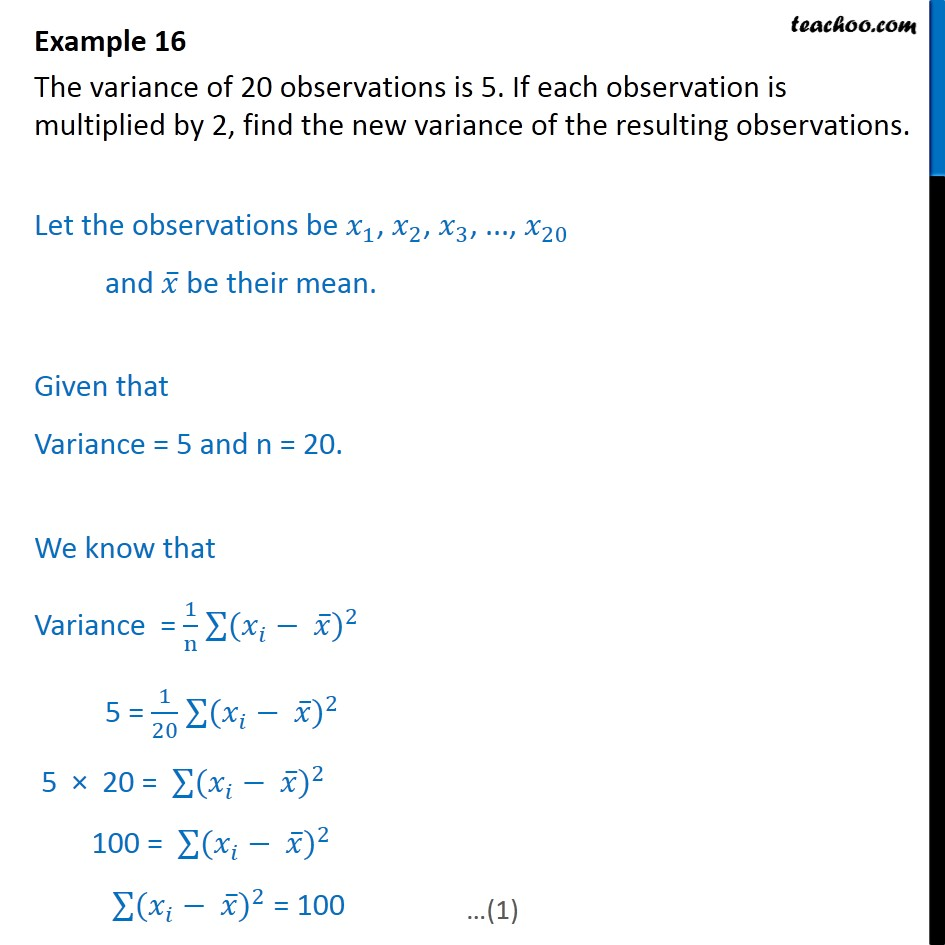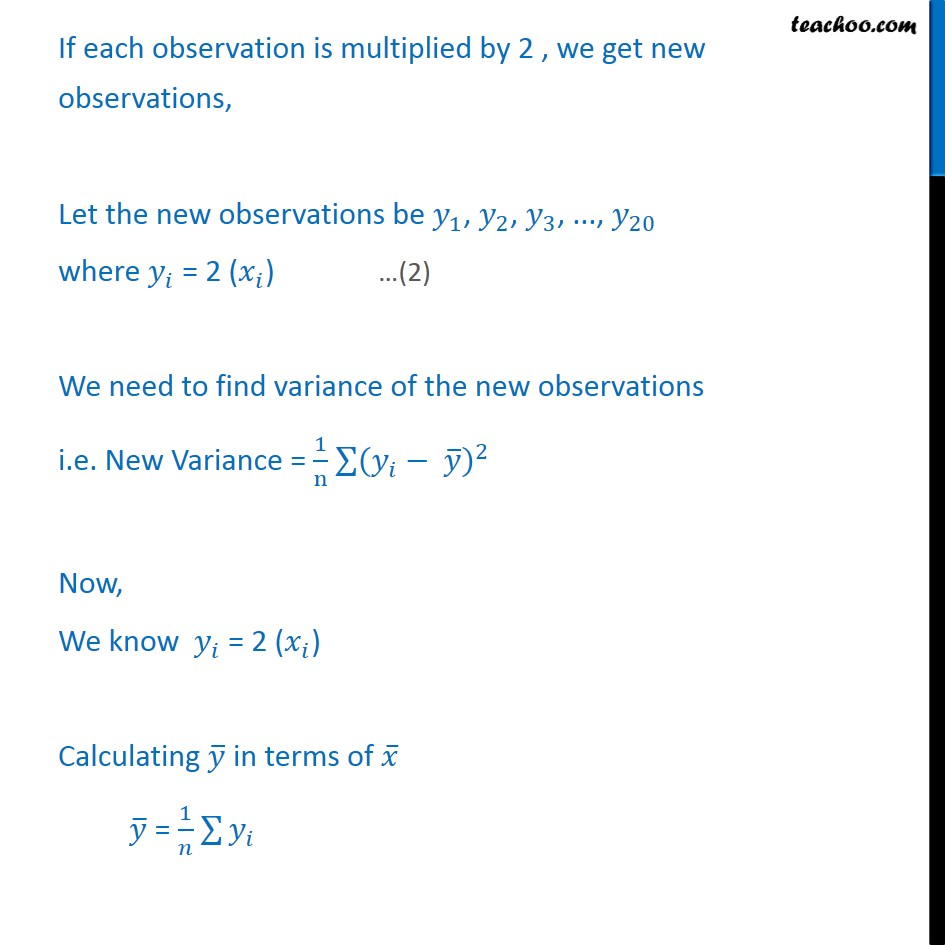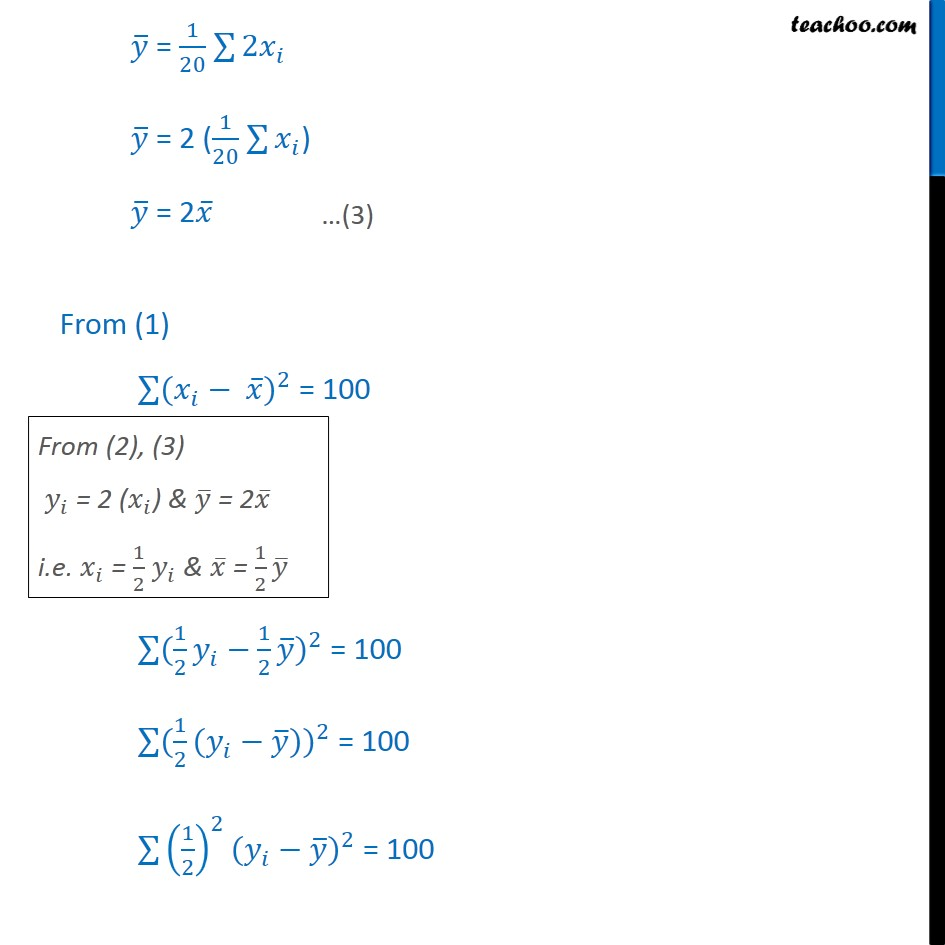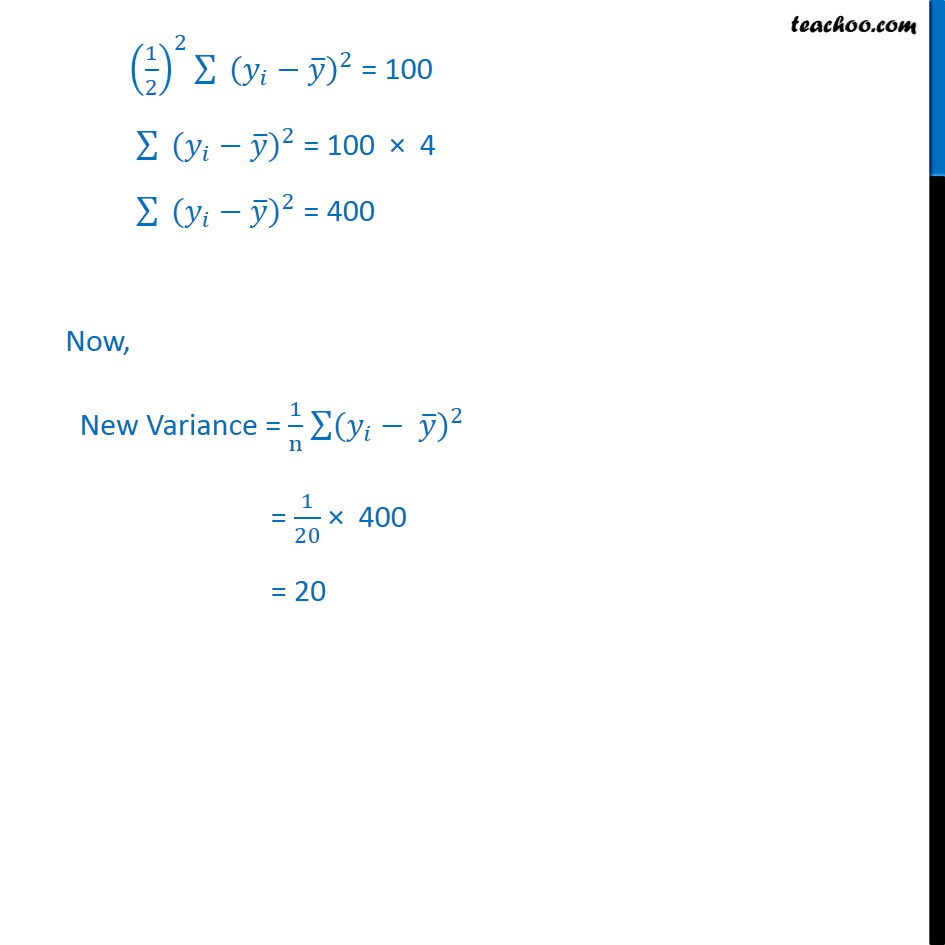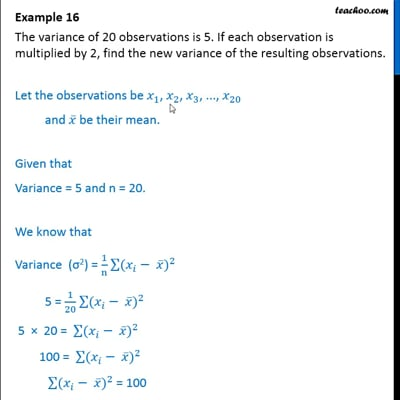This video is only available for Teachoo black users

### Transcript

Example 16 The variance of 20 observations is 5. If each observation is multiplied by 2, find the new variance of the resulting observations. Let the observations be 1 , 2 , 3 , ..., 20 and be their mean. Given that Variance = 5 and n = 20. We know that Variance = 1 n ( ) 2 5 = 1 20 ( ) 2 5 20 = ( ) 2 100 = ( ) 2 ( ) 2 = 100 If each observation is multiplied by 2 , we get new observations, Let the new observations be 1 , 2 , 3 , ..., 20 where = 2 ( ) We need to find variance of the new observations i.e. New Variance = 1 n ( ) 2 Now, We know = 2 ( ) Calculating in terms of = 1 = 1 20 2 = 2 ( 1 20 ) = 2 From (1) ( ) 2 = 100 ( 1 2 1 2 ) 2 = 100 ( 1 2 ( )) 2 = 100 1 2 2 ( ) 2 = 100 1 2 2 ( ) 2 = 100 ( ) 2 = 100 4 ( ) 2 = 400 Now, New Variance = 1 n ( ) 2 = 1 20 400 = 20